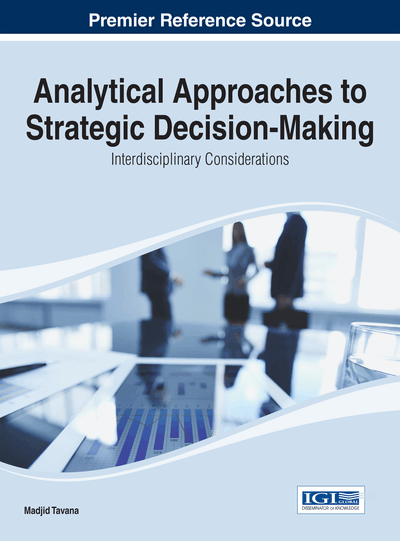# EOQ Model with Permissible Delay in Payments under Fuzzy Environment

Chandra K. Jaggi (University of Delhi, India), Anuj Sharma (University of Delhi, India) and Reena Jain (University of Delhi, India)
DOI: 10.4018/978-1-4666-5958-2.ch014
Available
\$37.50
No Current Special Offers

## Abstract

This chapter introduces an economic order quantity inventory model under the condition of permissible delay in payments in fuzzy environment. All the parameters of the model, excluding permissible delay period and cycle length, are taken to be trapezoidal Fuzzy numbers. The arithmetic operations are defined under the function principle. The cost function has been defuzzified using signed distance method and thereby solved to obtain the optimal replenishment period. The numerical example is presented to show the validity of the model followed by sensitivity analysis.
Chapter Preview
Top

## Introduction

In classical inventory analysis, it was tacitly assumed that the supplier is paid for the items as soon as the retailer receives the items. However, this assumption is not always fit in the real life situation, as the most of the supplier offer a certain fixed credit period for settle the account for stimulating retailer’s demand. During this credit period the retailer can start to accumulate revenues on the sales and earn interest on that revenue. But after this period, the supplier charges interest. Due to this period the total cost will be reduce. On the other hand, trade credit allowed by the supplier encourages the retailer to buy more and it is also a powerful promotional tool that attracts new retailers, whereas retailer takes it as an alternative incentive policy to quantity discounts. This implies that trade credit can play a major role in inventory control for both the supplier and retailer.

The series of theory to obtain the economic order policy, such that the relevant total cost (or profit) has a minimum (or maximum) value in crisp inventory models. But, most of the methods developed are assumed that all of the time parameters and relevant data are exactly known and fixed. Whereas, in real life, these assumptions seems to be impractical since they are generally vague and imprecise, even impossible to get the exact value, which implies the uncertainty in real world. In 1965, Zadeh introduced the concept of fuzzy set theory to address these problems. In 1978, Dubois and Prade defined any of the fuzzy numbers as a fuzzy subset of the real line, they presented that the Fuzzy numbers allow us to make the mathematical model of linguistic variable for fuzzy environment.

In the unstable environments it would not be easy to determine the exact value of the parameters demand rate, holding cost, ordering cost, unit cost, selling price, interest earned and interest paid by the decision maker. Thus the decision maker assumed all these parameters in fuzzy environment in order to address the realistic problem.

In this chapter fuzzified all parameters excluding cycle length and permissible delay period of Goyal (1985) namely demand, holding, ordering, unit cost, selling price, interest earned and interest paid as trapezoidal fuzzy numbers. The arithmetic operations are defined under the function principle. The total cost function and order quantity, has been defuzzified using signed distance method, in order to obtain the optimal order quantity.

## Complete Chapter List

Search this Book:
Reset